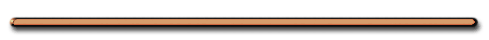Try this at home!

| Onsite |

Video Instruction

showmethephysics.com

D) Law of Conservation of Energy - unless work is done on or by a system, its total mechanical energy does not change.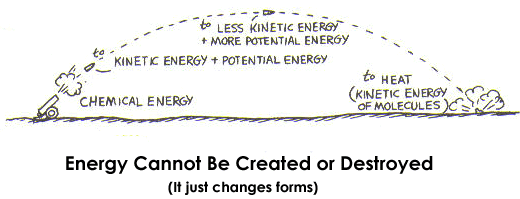Was energy created in this video?

Girl goes flying After the biggest piggy back blob jump

BlobLaunch.mov

The massive PE of the men on the platform completely turned into KE at the bottom of their jump.

The boy on the blob has more KE but less PE than the man.

1. Total Mechanical Energy

= PE + KE

Ex 1) Falling object (no friction)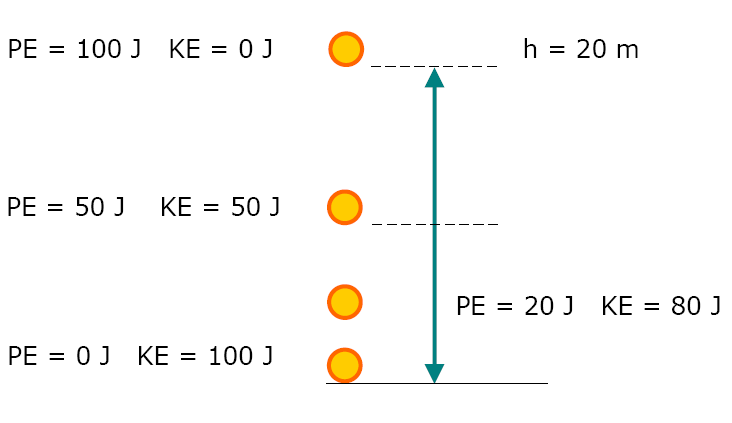As the Object Falls,

PE Lost = ....

...KE gained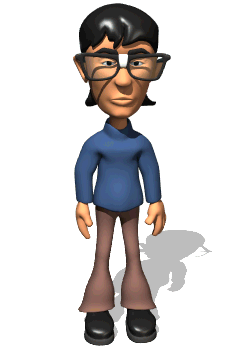Predict how far up the incline each ball will go. (assume no friction)Why does each ball rise to the same height  from which it began?PE depends only on height. Ball stops when  all KE turns into PE- Teachers DomainEx 2)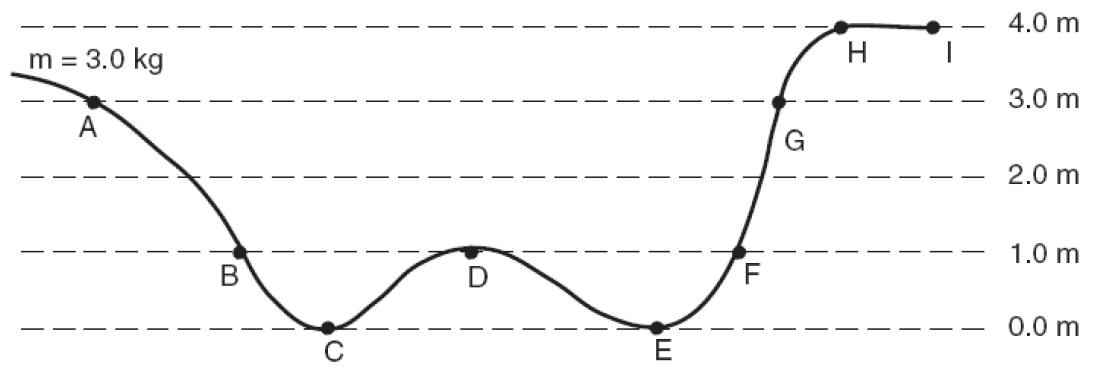IF KE at A = 0

PE = ?

PE = mgh,

g = 10 m/s2PE = 3 kg(10 m/s2)3 m

= 90 J

 PE KE A 90 J 0B?m = 3 kg, h = 1 m

 B PE KE 30 J 60 JC?

m = 3 kg, h = 0

 C PE KE 0 J (h = 0) 90 JD?

m = 3 kg, h = 1 m

 D PE KE 30 J 60 Jm = 3 kg, h = 0

 E PE KE 0 J (h = 0) 90 Jm = 3 kg, h = 1 m

 F PE KE 30 J 60 Jm = 3 kg, h = 3 m

 G PE KE 90 J 0 JH?

 H Never gets there! Doesn't have the energy to go any higher than GPhysics of Designing RollercoastersAt what minimum height should you place the ball so it makes it all the way around the loop?

UC Irvine

School blocks YouTube? Use the files below.The ball has to be placed at a height above the top of the loop so it has the KE to continue.